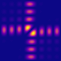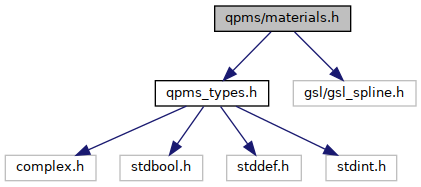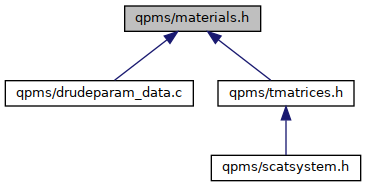QPMS Electromagnetic multiple scattering library and toolkit.
materials.h File Reference

Optical properties of materials. More...

#include "qpms_types.h"
#include <gsl/gsl_spline.h>
Include dependency graph for materials.h:This graph shows which files directly or indirectly include this file:Go to the source code of this file.

## Data Structures

struct  qpms_epsmu_generator_t
Prototype for general optical property generator for isotropic materials. More...

struct  qpms_ldparams_triple_t
A $$(f_j, \omega_j, \gamma_j)$$ triple for qpms_ldparams_t. More...

struct  qpms_ldparams_t
Structure holding Lorentz-Drude model parameters of a material. More...

struct  qpms_permittivity_interpolator_t
Interpolator of tabulated optical properties. More...

## Macros

#define SPEED_OF_LIGHT   (2.99792458e8)
Speed of light in m/s.

## Typedefs

typedef struct qpms_epsmu_generator_t qpms_epsmu_generator_t
Prototype for general optical property generator for isotropic materials.

typedef struct qpms_ldparams_triple_t qpms_ldparams_triple_t
A $$(f_j, \omega_j, \gamma_j)$$ triple for qpms_ldparams_t.

typedef struct qpms_ldparams_t qpms_ldparams_t
Structure holding Lorentz-Drude model parameters of a material. More...

typedef struct qpms_permittivity_interpolator_t qpms_permittivity_interpolator_t
Interpolator of tabulated optical properties.

## Functions

static qpms_epsmu_t qpms_epsmu_generator_eval (qpms_epsmu_generator_t gen, complex double omega)
Convenience function for generating material properties at given frequency.

qpms_epsmu_t qpms_epsmu_const_g (complex double omega, const void *epsmu)
Constant optical property "generator" for qpms_epsmu_generator_t. More...

static complex double qpms_refindex (qpms_epsmu_t em)
Gets refractive index of a material from its permeability and permittivity. More...

static complex double qpms_wavenumber (complex double omega, qpms_epsmu_t em)
Gets wave number k from angular frequency and material permeability and permittivity. More...

static complex double qpms_waveimpedance (qpms_epsmu_t em)
Gets (relative) wave impedance $$\eta_r$$ from material permeability and permittivity. More...

complex double qpms_lorentzdrude_eps (complex double omega, const qpms_ldparams_t *)
Lorentz-Drude permittivity.

qpms_epsmu_t qpms_lorentzdrude_epsmu (complex double omega, const qpms_ldparams_t *)
Lorentz-Drude optical properties, with relative permeability set always to one.

qpms_epsmu_t qpms_lorentzdrude_epsmu_g (complex double omega, const void *ldparams)
Lorentz-Drude optical properties, with relative permeability set always to one, compatible with qpms_epsmu_generator_t. More...

qpms_permittivity_interpolator_tqpms_permittivity_interpolator_create (const size_t incount, const double *wavelength_m, const double *n, const double *k, const gsl_interp_type *iptype)
Creates a permittivity interpolator from tabulated wavelengths, refraction indices and extinction coeffs. More...

qpms_permittivity_interpolator_tqpms_permittivity_interpolator_from_yml (const char *path, const gsl_interp_type *iptype)
Creates a permittivity interpolator from an yml file downloaded from refractiveindex.info website. More...

complex double qpms_permittivity_interpolator_eps_at_omega (const qpms_permittivity_interpolator_t *interp, double omega_SI)
Evaluates interpolated material permittivity at a given angular frequency.

qpms_epsmu_t qpms_permittivity_interpolator_epsmu_g (complex double omega, const void *interpolator)
Evaluates interpolated material permittivity at a given angular frequency, qpms_epsmu_generator_t compatible version. More...

double qpms_permittivity_interpolator_omega_min (const qpms_permittivity_interpolator_t *ip)
Returns the minimum angular frequency supported by the interpolator.

double qpms_permittivity_interpolator_omega_max (const qpms_permittivity_interpolator_t *ip)
Returns the minimum angular frequency supported by the interpolator.

void qpms_permittivity_interpolator_free (qpms_permittivity_interpolator_t *interp)
Destroy a permittivity interpolator.

static complex double qpms_drude_epsilon (complex double eps_inf, complex double omega_p, complex double gamma_p, complex double omega)
Relative permittivity from the Drude model. More...

## Variables

const qpms_ldparams_t *const QPMS_LDPARAMS_AG
Lorentz-Drude parameters from [rakic_optical_1998] for silver.

const qpms_ldparams_t *const QPMS_LDPARAMS_AU
Lorentz-Drude parameters from [rakic_optical_1998] for gold.

const qpms_ldparams_t *const QPMS_LDPARAMS_CU
Lorentz-Drude parameters from [rakic_optical_1998] for copper.

const qpms_ldparams_t *const QPMS_LDPARAMS_AL
Lorentz-Drude parameters from [rakic_optical_1998] for aluminium.

const qpms_ldparams_t *const QPMS_LDPARAMS_CR
Lorentz-Drude parameters from [rakic_optical_1998] for chromium.

const qpms_ldparams_t *const QPMS_LDPARAMS_TI
Lorentz-Drude parameters from [rakic_optical_1998] for titanium.

const qpms_ldparams_t *const QPMS_LDPARAMS_BE
Lorentz-Drude parameters from [rakic_optical_1998] for beryllium.

const qpms_ldparams_t *const QPMS_LDPARAMS_NI
Lorentz-Drude parameters from [rakic_optical_1998] for nickel.

const qpms_ldparams_t *const QPMS_LDPARAMS_PD
Lorentz-Drude parameters from [rakic_optical_1998] for polonium.

const qpms_ldparams_t *const QPMS_LDPARAMS_PT
Lorentz-Drude parameters from [rakic_optical_1998] for platinum.

const qpms_ldparams_t *const QPMS_LDPARAMS_W
Lorentz-Drude parameters from [rakic_optical_1998] for tungsten.

## Detailed Description

Optical properties of materials.

## ◆ qpms_ldparams_t

 typedef struct qpms_ldparams_t qpms_ldparams_t

Structure holding Lorentz-Drude model parameters of a material.

$\varepsilon = \varepsilon_\infty + \sum_j=0^{n-1} \frac{f_j \omega_p^2}{\omega_j^2-\omega^2+i\omega\gamma_j}$

## ◆ qpms_drude_epsilon()

 static complex double qpms_drude_epsilon ( complex double eps_inf, complex double omega_p, complex double gamma_p, complex double omega )
inlinestatic

Relative permittivity from the Drude model.

Parameters
 eps_inf Relative permittivity "at infinity". omega_p Plasma frequency $$\omega_p$$ of the material. gamma_p Decay constant $$\gamma_p$$ of the material. omega Frequency $$\omega$$ at which the permittivity is evaluated.

## ◆ qpms_epsmu_const_g()

 qpms_epsmu_t qpms_epsmu_const_g ( complex double omega, const void * epsmu )

Constant optical property "generator" for qpms_epsmu_generator_t.

Parameters
 omega Frequency ignored. epsmu Points to the qpms_epsmu_t to be returned.

## ◆ qpms_lorentzdrude_epsmu_g()

 qpms_epsmu_t qpms_lorentzdrude_epsmu_g ( complex double omega, const void * ldparams )

Lorentz-Drude optical properties, with relative permeability set always to one, compatible with qpms_epsmu_generator_t.

Parameters
 ldparams Lorentz-Drude parameters, in reality const qpms_ldparams_t *.

## ◆ qpms_permittivity_interpolator_create()

 qpms_permittivity_interpolator_t* qpms_permittivity_interpolator_create ( const size_t incount, const double * wavelength_m, const double * n, const double * k, const gsl_interp_type * iptype )

Creates a permittivity interpolator from tabulated wavelengths, refraction indices and extinction coeffs.

Parameters
 wavelength_m Tabulated vacuum wavelength in metres, in strictly increasing order. n Tabulated refraction indices at omega. k Tabulated extinction coefficients. iptype GSL interpolator type

## ◆ qpms_permittivity_interpolator_epsmu_g()

 qpms_epsmu_t qpms_permittivity_interpolator_epsmu_g ( complex double omega, const void * interpolator )

Evaluates interpolated material permittivity at a given angular frequency, qpms_epsmu_generator_t compatible version.

Permeability is always set to one. Imaginary part of omega is discarded.

Parameters
 omega Angular frequency. The imaginary part is ignored! interpolator Interpolator of type qpms_permittivity_interpolator_t

## ◆ qpms_permittivity_interpolator_from_yml()

 qpms_permittivity_interpolator_t* qpms_permittivity_interpolator_from_yml ( const char * path, const gsl_interp_type * iptype )

Creates a permittivity interpolator from an yml file downloaded from refractiveindex.info website.

Parameters
 path Path to the yml file. iptype GSL interpolator type

## ◆ qpms_refindex()

 static complex double qpms_refindex ( qpms_epsmu_t em )
inlinestatic

Gets refractive index of a material from its permeability and permittivity.

$n = \sqrt{\mu_r \varepsilon_r}$

## ◆ qpms_waveimpedance()

 static complex double qpms_waveimpedance ( qpms_epsmu_t em )
inlinestatic

Gets (relative) wave impedance $$\eta_r$$ from material permeability and permittivity.

\eta_r = \sqrt{\mu_r / \varepsilon_r} \f]

## ◆ qpms_wavenumber()

 static complex double qpms_wavenumber ( complex double omega, qpms_epsmu_t em )
inlinestatic

Gets wave number k from angular frequency and material permeability and permittivity.

$k = \frac{n\omega}{c_0} = \frac{\omega\sqrt{\mu_r \varepsilon_r}}{c_0}$